# GRE Subject Test: Physics : Energy and Momentum

## Example Questions

### Example Question #2 : Special Relativity

A relativistic particle of mass has a total energy 37 times its rest energy.  What is the momentum of the particle, in units of mc?

52

37

144

98

21

37

Explanation:

The total energy of a relativistic particle is related to its rest mass energy Eo by: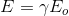Where gamma is related to the momentum by: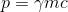Combining the equations and solving for p, we get: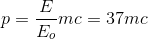Which, in the units specified, is 37.

### Example Question #1 : Special Relativity

A particle of mass m traveling at a relativistic speed has a momentum of 50 mc.  What is the total energy of the particle, expressed in units of the rest mass energy Eo?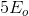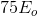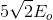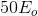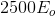Explanation:

For a relativistic particle, momentum is given by:from which we can solve for gamma: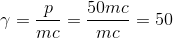Total energy of a relativistic particle is given by: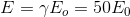### Example Question #1 : Energy And Momentum

The rest mass energy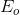of a particle with massis one quarter of its total energy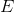. What is the of the particle's momentum, in units of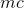?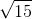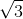Explanation:

The question tells us: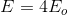Where the rest mass energy of a particle is: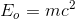Using the equation for the total energy of a particle, we can substitute: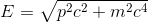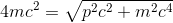Solving this for, we find: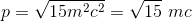Which, in units of mc, gives us the correct answer.

### Example Question #1 : Energy And Momentum

The relativistic momentum of a particle with massis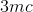.  What is the total energyof the particle, given in units of the rest mass energy?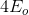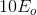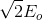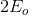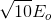Explanation:

The total energy of a relativistic particle is given by:Substituting the momentum, we get: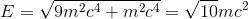Because the rest mass energy of a particle is given by:The total energy is: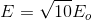### Example Question #2 : Special Relativity

A scientist measures the spectrum of relativistic jet emitted from a black hole. He finds that the a particular spectral line, which has a stationary wavelength of 212.5 nm, has a Doppler shifted wavelength of 643.7 nm. What is the radial velocity of the relativistic jet?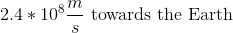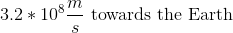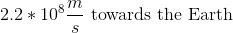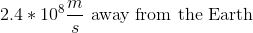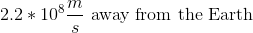Explanation:

The relativistic Doppler shift equation is given by: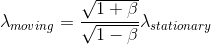Where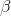is defined as: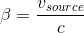Because the stationary wavelength is shorter than the moving wavelength, the object must be receding from the Earth, eliminating two answers.

The speed of light is approximately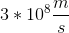, so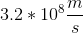is not a possible answer.

Making the approximation that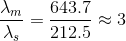Combining this with the first equation: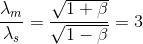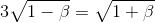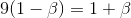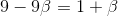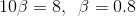From beta, we can find the velocity: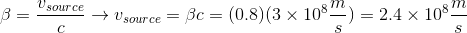### Example Question #1 : Energy And Momentum

A particle in an accelerator has a total energy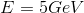and a relativistic momentum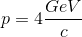. What is the rest mass of the particle, in units of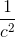?Explanation:

The total energy of a relativistic particle is given by:Solving for the rest mass: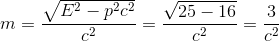Which, in the units specified, gives the answer 3.

### All GRE Subject Test: Physics Resources